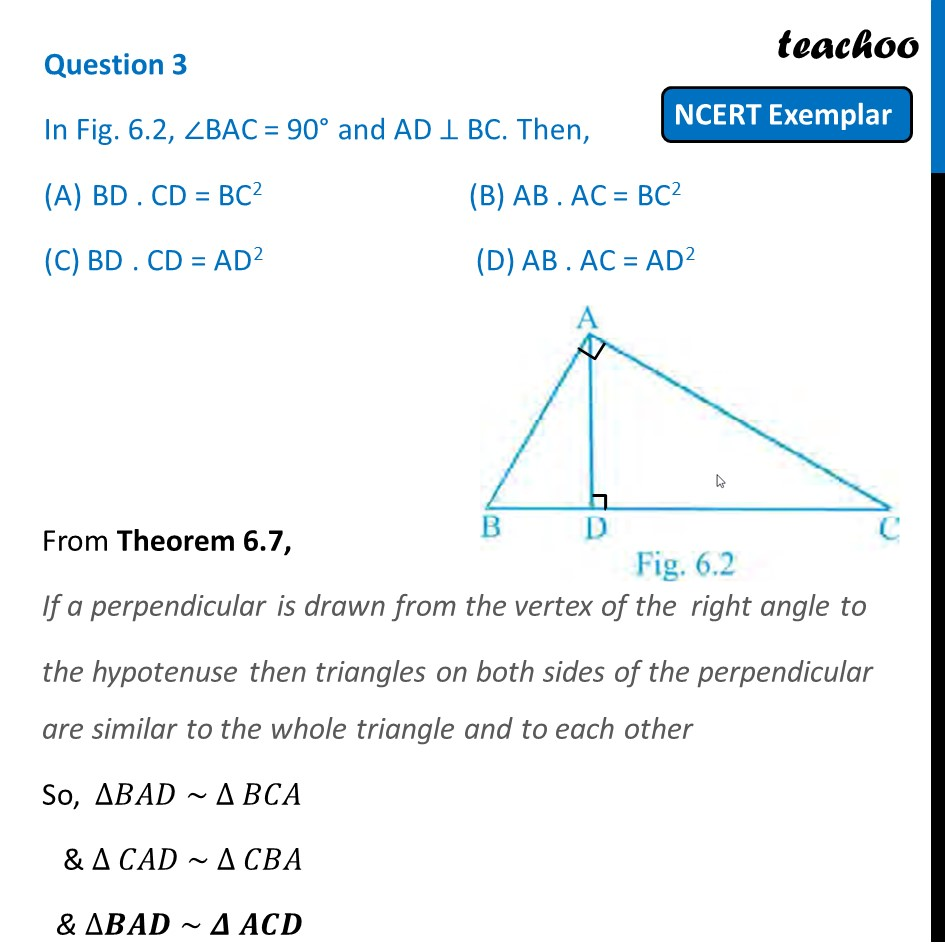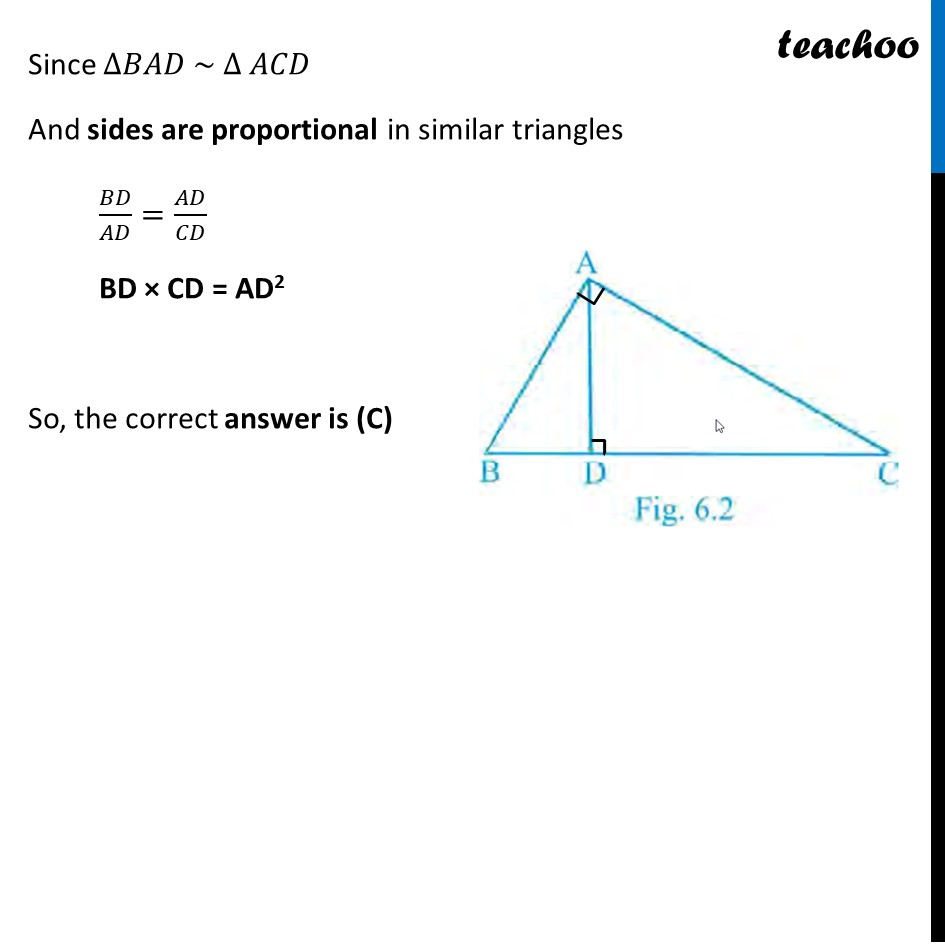## (D) AB . AC = AD 2

This question is inspired from  Example 10 - Chapter 6 Class 10 - Triangles1. Chapter 6 Class 10 Triangles (Term 1)
2. Serial order wise
3. NCERT Exemplar - MCQ

Transcript

Question 3 In Fig. 6.2, ∠BAC = 90° and AD ⊥ BC. Then, BD . CD = BC2 (B) AB . AC = BC2 (C) BD . CD = AD2 (D) AB . AC = AD2 From Theorem 6.7, If a perpendicular is drawn from the vertex of the right angle to the hypotenuse then triangles on both sides of the perpendicular are similar to the whole triangle and to each other So, ∆𝐵𝐴𝐷 ~ ∆ 𝐵𝐶𝐴 & ∆ 𝐶𝐴𝐷 ~ ∆ 𝐶𝐵𝐴 & ∆𝑩𝑨𝑫 ~ 𝜟 𝑨𝑪𝑫 From Theorem 6.7, If a perpendicular is drawn from the vertex of the right angle to the hypotenuse then triangles on both sides of the perpendicular are similar to the whole triangle and to each other So, ∆𝐵𝐴𝐷 ~ ∆ 𝐵𝐶𝐴 & ∆ 𝐶𝐴𝐷 ~ ∆ 𝐶𝐵𝐴 & ∆𝑩𝑨𝑫 ~ 𝜟 𝑨𝑪𝑫 Since ∆𝐵𝐴𝐷 ~ Δ 𝐴𝐶𝐷 And sides are proportional in similar triangles 𝐵𝐷/𝐴𝐷=𝐴𝐷/𝐶𝐷 BD × CD = AD2 So, the correct answer is (C)